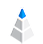# The Correlation Coefficient Demystified

Correlation and the correlation coefficient seem to be difficult to understand. They sound like some weird mathematical, statistical thing. However, once you understand them, you will think in a totally new way about causality and how things are related in all aspects of life. Read this article and find out how Pearson and Spearman changed statistics.

## What Is the Correlation Coefficient?

The correlation coefficient is a metric that helps measure the strength of the relationship between two numerical datasets. For example, you may have a list of students and know their ages and heights. You can then ask what the correlation is between age and height. It is likely that in most cases, the taller a student is, the older she/he is, and vice versa if someone is rather old, you can guess that she/he is tall. Of course, this correlation does not exist among full-grown adults.

Simply speaking, correlation mean that the bigger (or more) something is, the bigger (or more) something else is.If the absolute value of the calculated correlation coefficient is high, then the connection between the variables is strong. If the coefficient is low, there might be only a weak connection or maybe no relationship at all.

A negative correlation coefficient means reverse correlation that is, the bigger (or more) something is, the smaller (or less) something else is.

As a rule of thumb, you can use this table:

Relationship Applies To Correlation Coefficient
All Cases Perfect 1
Almost All Cases Almost Perfect 0.9-1
Most Cases Very Strong 0.8-0.9
Many Cases Strong 0.7-0.8
Some Cases Moderate 0.5-0.7
A Few Cases Weak 0.3-0.5
Few Cases Very Weak 0.2-0.3
Very Few Cases Negligible Below 0.2

## Different Correlation Algorithms

There are many different algorithms for calculating correlation, and each one has different properties and variants. Pearson is the most popular, but I would suggest Spearman because it has fewer limitations and can be applied more widely.

### Pearson Correlation

https://en.wikipedia.org/wiki/Pearson_product-moment_correlation_coefficient

Inventor: Karl Pearson ~ 1895

Other names: Pearson Product-Moment Correlation Coefficient, PPMCC, PCC, Pearson’s r

Population coefficient is denoted by: Greek letter ρ (rho)

Sample coefficient is denoted by: r

Good for:

• If you care about the amount of growth
• If you also want to calculate the confidence interval
• If you have no outliers at all Pearson (unlike Spearman) is very sensitive to outliers
• If you want to check linear association (not good for nonlinear relationships)

Formula:### Spearman Correlation

https://en.wikipedia.org/wiki/Spearman%27s_rank_correlation_coefficient

Inventor: Charles Spearman ~ 1904

Other names: Spearman’s Rank Correlation Coefficient, Spearman’s rho

Coefficient is denoted by: Greek letter ρ (rho)

Good for:

• If outliers exist Spearman (unlike Pearson) is not sensitive to outliers
• If you also want to calculate the confidence interval
• If you want to find linear and nonlinear relationships
• If there are no repeated values (more identical x or y values)
• If you care about the relationship only not the amount of growth (Spearman only checks monotony)

Formula:### Kendall Correlation

https://en.wikipedia.org/wiki/Kendall_rank_correlation_coefficient

Inventor: Maurice Kendall ~ 1938

Other names: Kendall Rank Correlation Coefficient, Kendall’s tau Coefficient

Coefficient is denoted by: Greek letter τ (tau)

Good for:

• If outliers exist
• If you want to find linear and nonlinear relationships
• If repeated values exist
• If you do not want to calculate the confidence interval

Formula:A/B test calculator!

## Correlation Is Not Causation

It is very important not to forget that Correlation does not imply causation!

If you find a strong correlation in your data, the following relationships are possible:

• X causes Y (this is what most people incorrectly assume)
• Y causes X (this is what most people might incorrectly think)
• X and Y are consequences of a common cause (this is very frequent)
• X causes Y and Y causes X
• X causes Z which causes Y
• There is no connection between X and Y (it is just a coincidence)

If there is no mathematical correlation between variables, it does not mean that there is no relationship. There might be a strong connection, but other factors can be thecause so you see no correlation.

## What Is Correlation Good For?

• There are mathematical algorithms to filter out the effects of other variables, so you can find real relationships if you take into account many factors.
• If the correlation is strong, you can predict X from Y, and Y from X
• Based on the results of correlations, you can investigate your research further if you find surprisingly weak or strong correlations and the calculated coefficient conflicts with your hypothesis

## Try out our free online calculator

Correlation Test Calculator